# Circuit Ensures Smooth "Soft Start" for Isolated Converter, Limits In-Rush Current

## Texas Instruments LM5025

Mangjing Xie

Electronic Design

Isolated dc-dc converters are prone to excessive in-rush current, but a small soft-start circuit can solve the problem and limit that current.

Most dc-dc converters require a soft-start circuit to limit the in-rush current at startup. Although a smooth soft start is required for systems with power-on reset (POR), this is difficult to implement in an isolated converter with a controller on the primary side and a limited duty cycle or current.

Figure 1 shows the soft start of a forward converter with a duty-cycle soft start from the primary side. The steady-state output of the converter is 12 V. A 50% load current is applied at 10 V, which is the POR threshold of the system. As soon as a load is applied, the output drops and triggers system shutdown, causing the system power to cycle several times. At the end of soft start, the output overshoots 10%, which is not desirable.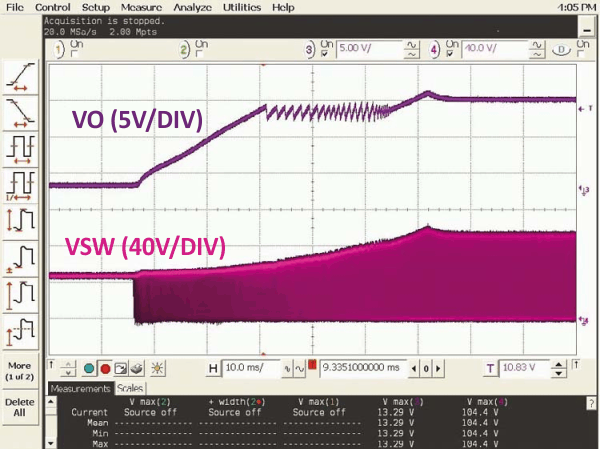Figure 1. The output of a forward converter during startup mode, with a load applied at 10 V, shows the system power-cycling and the output overshooting by about 10%.

A simple circuit can implement a smooth soft start for an isolated converter in a system using the LM5025 active-clamp, voltage-mode PWM controller. Figure 2 shows the concept of this secondary-side soft-start approach.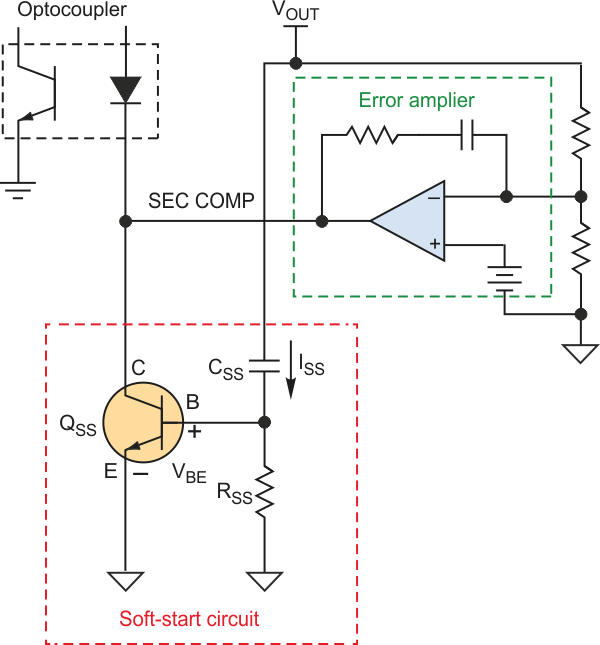Figure 2. The basic secondary-side soft-start approach adds an auxiliary circuit to the secondary-side compensation node.

When first applying the input, the converter output (VOUT) starts to rise, capacitor CSS is charging up, and the CSS charging current (ISS) flows through resistor RSS. When ISS is higher than VBE(ON)/RSS, QSS then turns on and starts to pull current from the secondary-side comp node (SEC COMP), thus reducing the duty cycle. During soft start, the error amplifier saturates and the soft-start circuit dominates the feedback loop. The converter, CSS, RSS, QSS, and optocoupler form a closed loop. When the output rises to regulation, the error amplifier starts to regulate and ISS goes down, thus turning QSS off.

Equation 1 shows the transfer function from VOUT to the optocoupler current: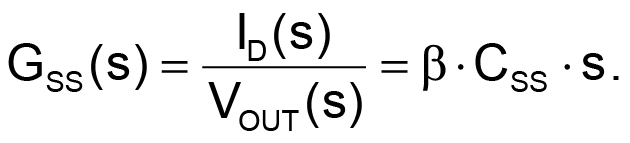(1)

While effective, this simple circuit might not be stable because the QSS forward gain β is high and varies dramatically from part to part. To stabilize this circuit, you need to insert a gain-reducing resistor RE between the emitter of QSS and ground (Fig. 3). Increasing RE can reduce the feedback-loop gain during startup.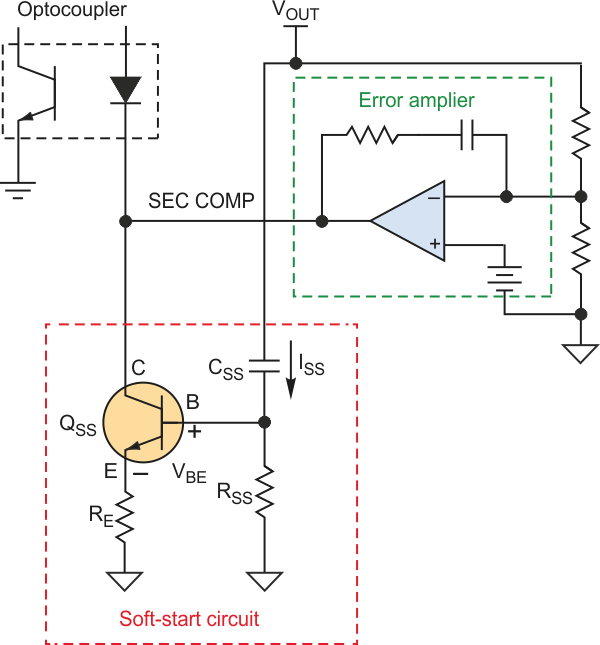Figure 3. Adding gain-stabilizing resistor RE stabilizes the soft-start circuit.

Equation 2 shows the soft-start circuit transfer function with RE: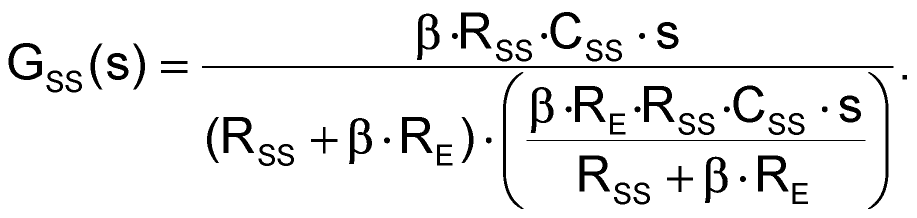(2)

At high frequencies, Equation 3 is an approximation of Equation 2: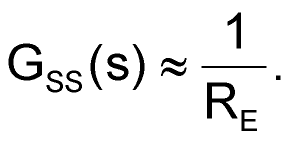(3)

For testing, the soft-start circuit to the converter was set up with CSS = 0.1 µF, RSS = 100 kΩ, and RE = 1.18 kΩ. Figure 4 shows the soft-start waveform with these circuit parameters. When the system starts pulling current, the soft-start circuit stops drawing current from the COMP and the duty cycle increases quickly. The converter continues to soft start after a minor dip caused by the load transient.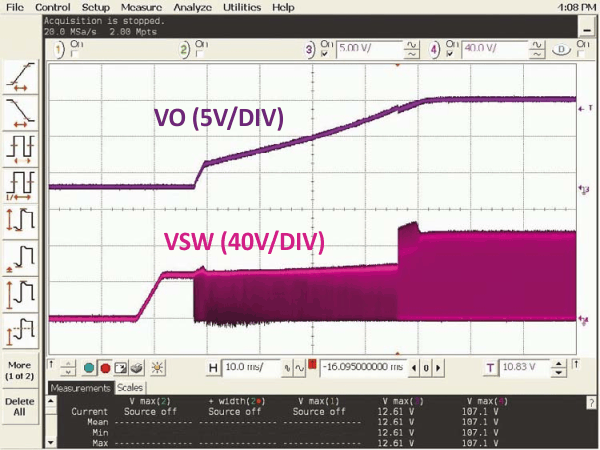Figure 4. The soft-start waveform shows the circuit stops drawing current from the COMP pin; as a result, the duty cycle increases and the converter continues the soft-start process.

The figure also shows that after the load is applied, the converter switching node (VSW) has an additional voltage spike. Figure 5 shows the zoomed-in waveform, which reveals that the system oscillates at 9.5 kHz.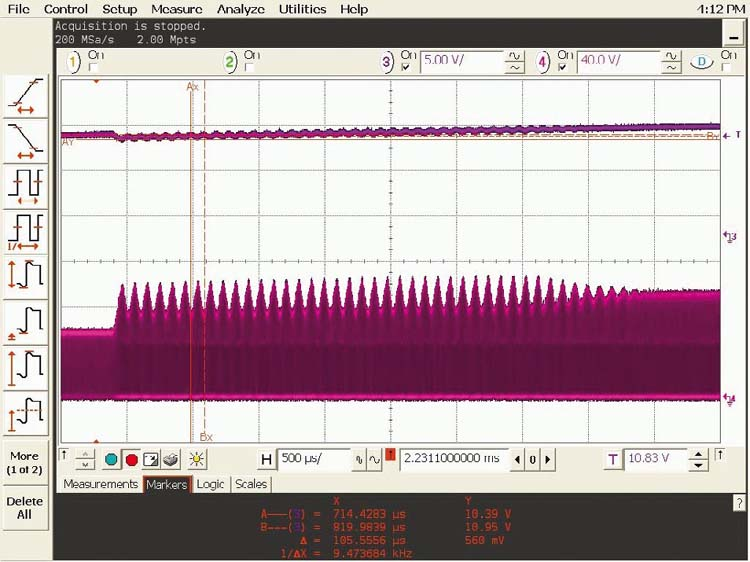Figure 5. The zoomed-in soft-start waveform shows system oscillation at 9.5 kHz, which isn’t acceptable.

The controller in this design is a voltage-mode controller. The power stage has 180° of phase drop because of the double poles. It’s necessary to add a zero to improve stability, done by adding capacitor CE in parallel with RE(Fig. 6). In order to add 45° to the phase margin, a zero was placed at 9.5 kHz (the measured oscillation frequency). With RE = 1.18 kΩ, this required a 15-nF capacitor.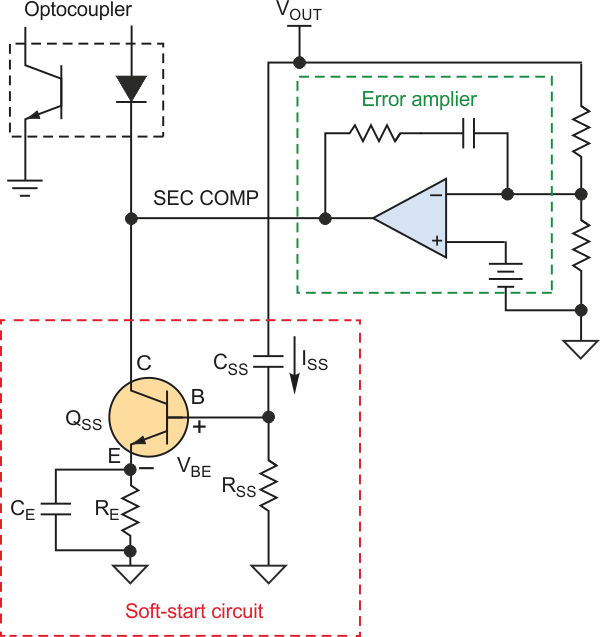Figure 6. Adding a zero improves stability, here done by placing capacitor CE in parallel with RE to add 45° to the phase margin.

Equation 4 shows the soft-start circuit transfer function with RE and CE in parallel: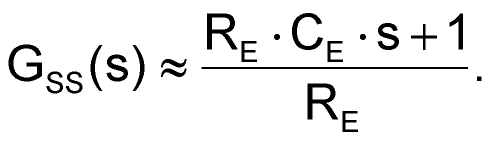(4)

Figure 7 shows the startup waveform with CE = 15 nF. Note that the oscillation is eliminated, while the total soft-start time is 50 ms.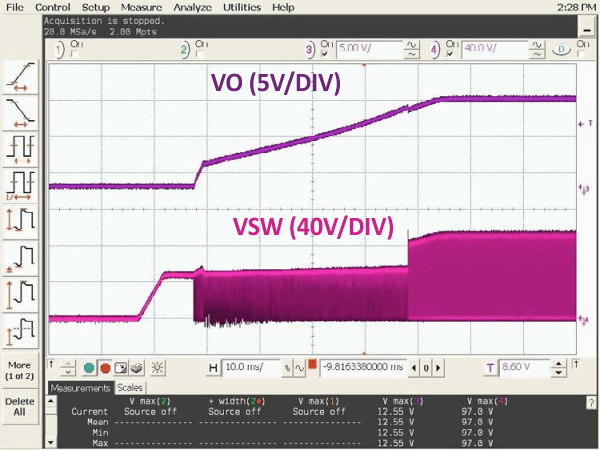Figure 7. Adding the zero via the small additional capacitance eliminates the oscillation;the final circuit has an overall soft-start time of 50 ms.

During soft start, the typical optocoupler diode current (IOPTO_D) is between 1.2 and 0.8 mA, which is determined by the LM5025 and the optocoupler forward gain. With RE = 1.18 kΩ, the voltage across RSS isSince VBE(ON) = 0.7 V, you can calculate ISS as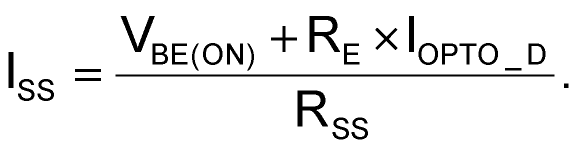ISS/CSS sets the output VOUT, dv/dt. To ensure the effectiveness of the secondary-side soft start, the primary-side soft start should be set to be much faster than the secondary-side soft start.

## Materials on the topic

electronicdesign.com